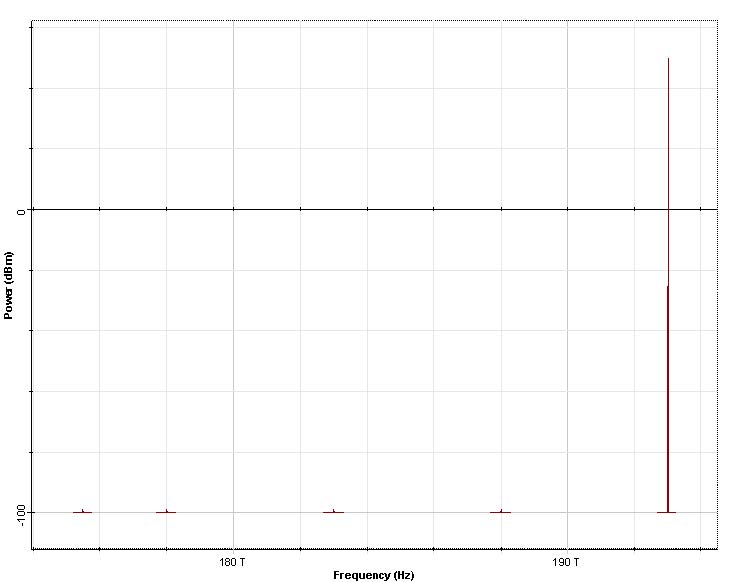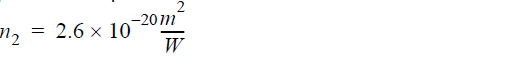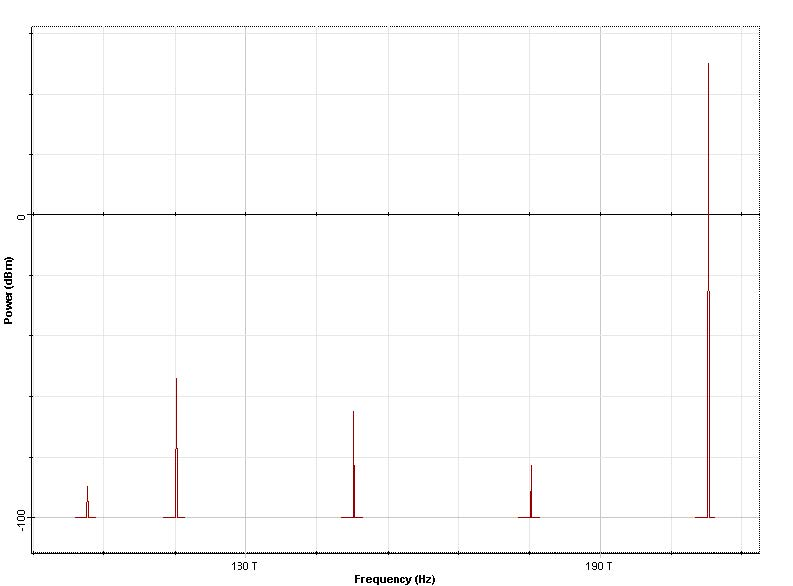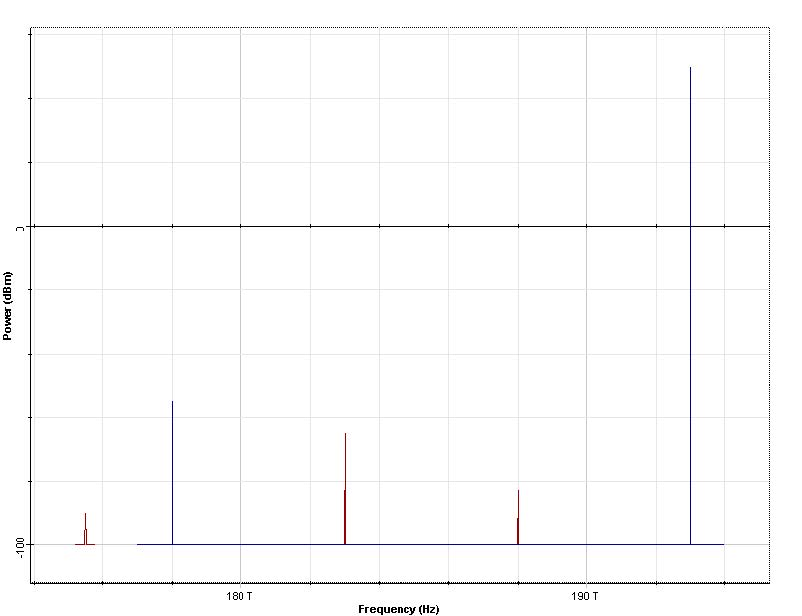The purpose of this lesson is to demonstrate light amplification resulting from the stimulated Raman scattering effect.

The layout with its global parameters is shown in Figure 1

Figure 1: Layout and global parameters

The input spectrum (Figure 2) consists of a strong (pump) wave at 193 THz (100W) and four weak (probe) waves at frequencies 188 THz, 183 THz, 178THz and 175.5THz. The powers of the probe-waves are all equal to -99dBm.Figure 2: Input spectrum

The fiber parameters are relevant to SMF-28 and are specified as “wavelength dependent” (from data files). Constant step-size is used with the maximum nonlinear phase shift equal to 5mrad and the nonlinear refractive index is:The fractional Raman contribution is ρ = 0.18.

The output spectrum (after 200m of propagation) is shown in Figure 3.Figure 3: Output spectrum

The gains obtained from Figure 3 are summarized in Table 1.
Table 1 Numerically obtained dependence of the gain on the probe frequency.

 Probe frequency [THz] Gain [dBm] 188 16.252 183 34.106 178 45.02 175.5 9.535

The pump power is 100W and the pump frequency is 193 THz. Fiber length is 200m.
On the other hand, the gain coefficient is given by:and using the fiber data, it is shown that the numerical results are in agreement (see table 2) with the formula in .

 Probe frequency [THz] Im( X1111 ( vp – vs ) ) Υs [ 1 / kmW ] G = 10log(exp(gP0L))[dBm] 188 4.045951e-001 1.28445 16.250 183 9.114571e-001 1.19659 34.103 178 1.251922e+000 1.149963 45.017 175.5 2.678715e-001 1.133812 9.496

Table 2: Analytically obtained results for the gain.Figure 4: Same asFigure 3, but the pump and one of the signals are converted to “parameterized”.

Note that the same results can be obtained in this using arbitrary configuration of sampled and parameterized signals. Figure 4 shows the same output spectrum (as Figure 3) however the pump and one of the signals are converted to parameterized signals. The numerical values of the gain for each signals (parameterized or sampled) are one and same. Calculations with parameterized signals are faster; however replacing the sampled signals with parameterized is not always possible.

Reference:

G. P. Agrawal, “Nonlinear fiber optics”, Academic press, 3rd edition, 2001 Chapter 8.